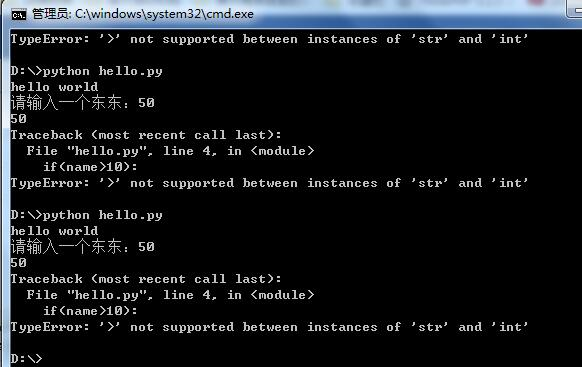python从头学1简单初步接触

python知道的很早，但是一直不想学，有抵触情绪，哈哈，不知道怎么的，总觉得没用大括号的语言都是异教徒。

print("hello world");
name = input("请输入一个东东：");
print(name);
if(name>10):
print("%d大于10"%(name));
else:
print("%d小于10"%name);#!/usr/bin/env python3
# -*- coding: utf-8 -*-
print("hello world");
name = input("请输入一个东东：");
print(name);
name = int(name);
if(name>=10):
print("%d大于等于10"%(name));
else:
print("%d小于10"%name);

print(10/3);
print(10//3);   #取整
print(10%3);    #取余

PING = '常量这样定义';
print(PING.encode('gb2312')) ;   #转换编码试试

table = [111,2222,3333,'试试看效果','瞎玩'];
print(table);
a = len(table);  #看长度
print(a);
table.append("尾部追加");
print(table);
table.insert(0,"插入第一");

print(table);

### tuple 和list不同好像主要是用()定义的，以后不能改了